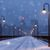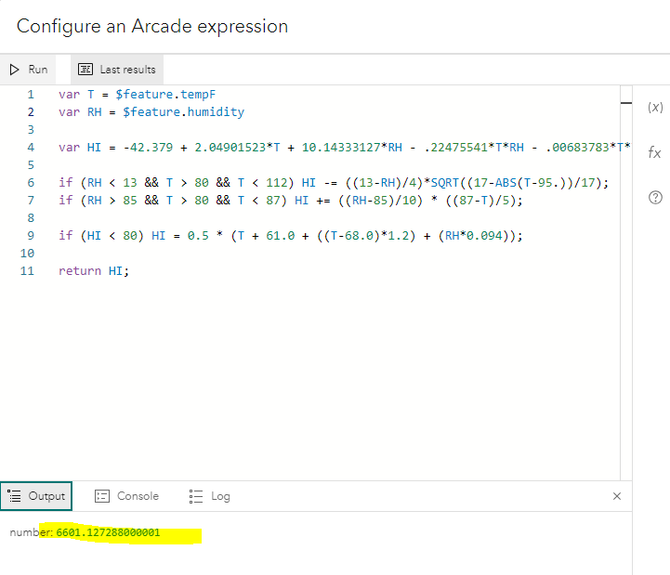172
4
10-02-2023 01:13 PMby
Occasional Contributor III

I have found this equation for Excel, but wondering if anybody has an expression in Arcade for °F?

A2 = Temperature in Celsius

B2 = Humidity

=IF((A2*9/5+32)<=80,A2,IF(AND(B2<13,((A2*9/5+32)>80),((A2*9/5+32)<112)),(((-42.379+2.04901523*(A2*9/5+32)+10.14333127*B2-0.22475541*(A2*9/5+32)*B2-0.00683783*(A2*9/5+32)*(A2*9/5+32)-0.05481717*B2*B2+0.00122874*(A2*9/5+32)*(A2*9/5+32)*B2+0.00085282*(A2*9/5+32)*B2*B2-0.00000199*(A2*9/5+32)*(A2*9/5+32)*B2*B2)-32)*5/9)-(((13-B2)/4)*SQRT((17-ABS((A2*9/5+32)-95))/17)),IF(AND(B2>85,((A2*9/5+32)>80),((A2*9/5+32)<87)),(((-42.379+2.04901523*(A2*9/5+32)+10.14333127*B2-0.22475541*(A2*9/5+32)*B2-0.00683783*(A2*9/5+32)*(A2*9/5+32)-0.05481717*B2*B2+0.00122874*(A2*9/5+32)*(A2*9/5+32)*B2+0.00085282*(A2*9/5+32)*B2*B2-0.00000199*(A2*9/5+32)*(A2*9/5+32)*B2*B2)-32)*5/9)+((B2-85)/10)*((87-(A2*9/5+32))/5),(((-42.379+2.04901523*(A2*9/5+32)+10.14333127*B2-0.22475541*(A2*9/5+32)*B2-0.00683783*(A2*9/5+32)*(A2*9/5+32)-0.05481717*B2*B2+0.00122874*(A2*9/5+32)*(A2*9/5+32)*B2+0.00085282*(A2*9/5+32)*B2*B2-0.00000199*(A2*9/5+32)*(A2*9/5+32)*B2*B2)-32)*5/9))))

1 Solution

Accepted SolutionsbyMVP Esteemed Contributor

This uses the formulas on the NOAA National Weather Service's Heat Index Equation page.

``````var T = 80; //Temperature in °F
var RH = 12; //Relative Humidity

var HI = -42.379 + 2.04901523*T + 10.14333127*RH - .22475541*T*RH - .00683783*T*T - .05481717*RH*RH + .00122874*T*T*RH + .00085282*T*RH*RH - .00000199*T*T*RH*RH;

if (RH < 13 && T > 80 && T < 112) HI -= ((13-RH)/4)*SQRT((17-ABS(T-95.))/17);
if (RH > 85 && T > 80 && T < 87) HI += ((RH-85)/10) * ((87-T)/5);

if (HI < 80) HI = 0.5 * (T + 61.0 + ((T-68.0)*1.2) + (RH*0.094));

return HI;``````

The calculated value can be checked  through the Heat Index Calculator page.

4 RepliesbyMVP Esteemed Contributor

This uses the formulas on the NOAA National Weather Service's Heat Index Equation page.

``````var T = 80; //Temperature in °F
var RH = 12; //Relative Humidity

var HI = -42.379 + 2.04901523*T + 10.14333127*RH - .22475541*T*RH - .00683783*T*T - .05481717*RH*RH + .00122874*T*T*RH + .00085282*T*RH*RH - .00000199*T*T*RH*RH;

if (RH < 13 && T > 80 && T < 112) HI -= ((13-RH)/4)*SQRT((17-ABS(T-95.))/17);
if (RH > 85 && T > 80 && T < 87) HI += ((RH-85)/10) * ((87-T)/5);

if (HI < 80) HI = 0.5 * (T + 61.0 + ((T-68.0)*1.2) + (RH*0.094));

return HI;``````

The calculated value can be checked  through the Heat Index Calculator page.by
Occasional Contributor III

@KenBuja Here is what I get when I input my fields:  6601.13byMVP Esteemed Contributor

What are the actual values for tempF and humidity? I tested this with several values for T and RH.by
Occasional Contributor III

@KenBuja My fault, it is correct.  I made an error on my part.  Thanks so much for putting the expression together!!!